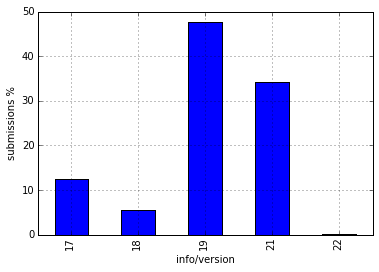### Fennec analysis¶

In :
import ujson as json
import matplotlib.pyplot as plt
import pandas as pd
import numpy as np
import plotly.plotly as py

from moztelemetry import get_pings, get_pings_properties, get_one_ping_per_client

%pylab inline

Populating the interactive namespace from numpy and matplotlib

In :
pings = get_pings(sc, app="Fennec", channel="release", submission_date="20150609", fraction=1)

In :
subset = get_pings_properties(pings, ["clientID", "info/manufacturer", "info/device", "info/version"])

In :
subset.filter(lambda x: x.get("clientID", None) is not None).count() # ClientID isn't present in release

Out:
0
In :
samsung = pd.DataFrame(subset.filter(lambda x: x["info/manufacturer"] == "samsung" and x["info/device"] == "GT-I9500").collect())
len(samsung)

Out:
4389
In :
histogram = samsung.groupby("info/version").apply(lambda x: len(x))

In :
(100*histogram/histogram.sum()).plot(kind="bar")
pylab.ylabel("submissions %")

Out:
<matplotlib.text.Text at 0x7f5ae463a150>In [ ]: